Középiskolai Matematikai és Fizikai Lapok
Informatika rovattal
 Már regisztráltál? Új vendég vagy?# Exercises and problems in PhysicsFebruary 2001

## New experimental problem:

M. 222. The angle of refraction of a puck bouncing back from a board is usually not identical to its angle of incidence. (The motion of a puck can be modelled using the lid of a jar.) How does the angle of refraction depend on the speed of the puck (lid) and on the angle of incidence? (6 points)

## New problems:

P. 3404. An aluminium ball with an empty interior floats in 20.0 oC water. The water with the ball in it is heated. Does the upthrust exerted onto the ball increase or decrease? Why? (3 points)

P. 3405. The springing of scooters is often carried out using the screw spring of varying pitch shown in the figure. What is the advantage of such a spring? (4 points)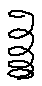P. 3406. How high does a rocket that is launched vertically at the first cosmic speed from the surface of the Earth go? (4 points)

P. 3407. There are three rods of identical size but different masses and homogeneous mass distribution placed atop each other in the arrangement shown from above in the figure. One end of each rod leans on a pillar, while the other end leans on the centre of another rod. The height of the pillars is identical. What is the force exerted by each pillar onto each rod? (Let m1=1 kg, m2=2 kg, m3=3 kg.) Does the result change when two rods are interchanged? (5 points)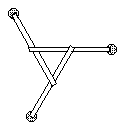P. 3408. The weight of an air-balloon inflated with air is measured using a very sensitive scale. The air is let out from the balloon and the weight of the balloon (and of the thread) is measured. What is the result? Why? (4 points)

P. 3409. A process made with an ideal gas is represented by a straight section on a (V,T) diagram. In what cases can the pressure at the middle of this section be the arithmetic mean of the respective pressure values of the initial and final states? (5 points)

P. 3410. There are such a homogeneous electric field E and a homogeneous magnetic field B perpendicular to the plane of the figure between the plates of a plane capacitor that positively charged particles shot parallel to the plates reach the screen without changing direction. The length of the plates of the plane capacitor is b and the screen is at distance c from the right-hand end of the plates. When the magnetic field is switched off the point where the charged particles hit the screen moves by y. Determine the specific charge Q/m of the particles. (Neglect the effect of gravitation.) (5 points)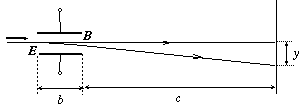P. 3411. A flat coil with N turns of radius r is placed at distance l from a long, straight conductor (l<<r). The flat coil and the straight conductor are in the same plane. A voltmeter is inserted into the long conductor, and the far ends of this latter are earthed. What does the voltmeter show when the current in the coil is uniformly increased from zero to I0 in time t0. (The self-induction of the straight conductor is negligible.) Data: N=100, l=100 cm, r=2 cm, t0=5 s, I0=2 A. (5 points)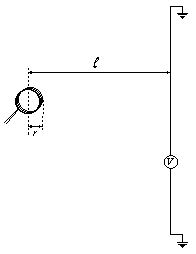P. 3412. Light does not get through perpendicular polar filters. When a third polar filter is slid between the first two some light may pass through. What is the maximum proportion of the incident light that can pass through such an arrangement? (5 points)

P. 3413. When one looks into the concave side of a shiny spoon, one sees oneself upside down. Turning the spoon and looking into its convex side, the image is erect and looks the same size as the inverted image seen in the concave side. Try this out and explain the phenomenon. (4 points)

P. 3414. At Paks Nuclear Power Plant the nucleus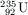, when hit by a thermal'' neutron, may e.g. split into a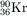and a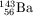, while three fast neutrons are also produced. The Kr and Ba isotopes are unstable and decay once more emitting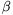radiation. What are the final products? (4 points)

P. 3415. A conventional turntable is tilted at angle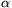. A small body is placed onto its disc, at distance r from its axis, at the lowest possible position. Then, starting from rest, the disc is turned at angular acceleration. The small body slips after time t. a) What is the coefficient of static friction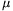between the small body and the disc? b) What is the required minimum value ofso that the small body does not slip at once at the beginning? (6 points)